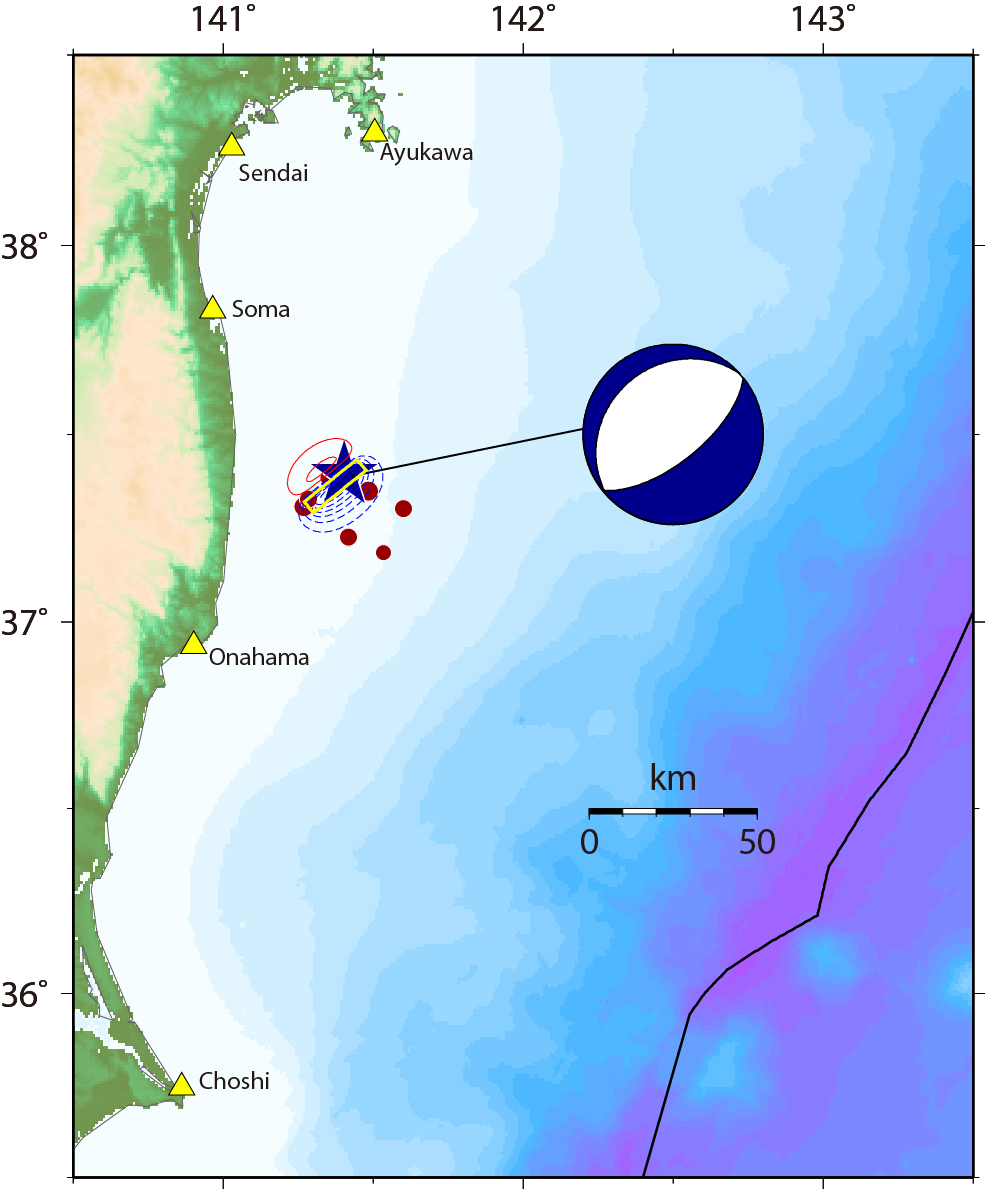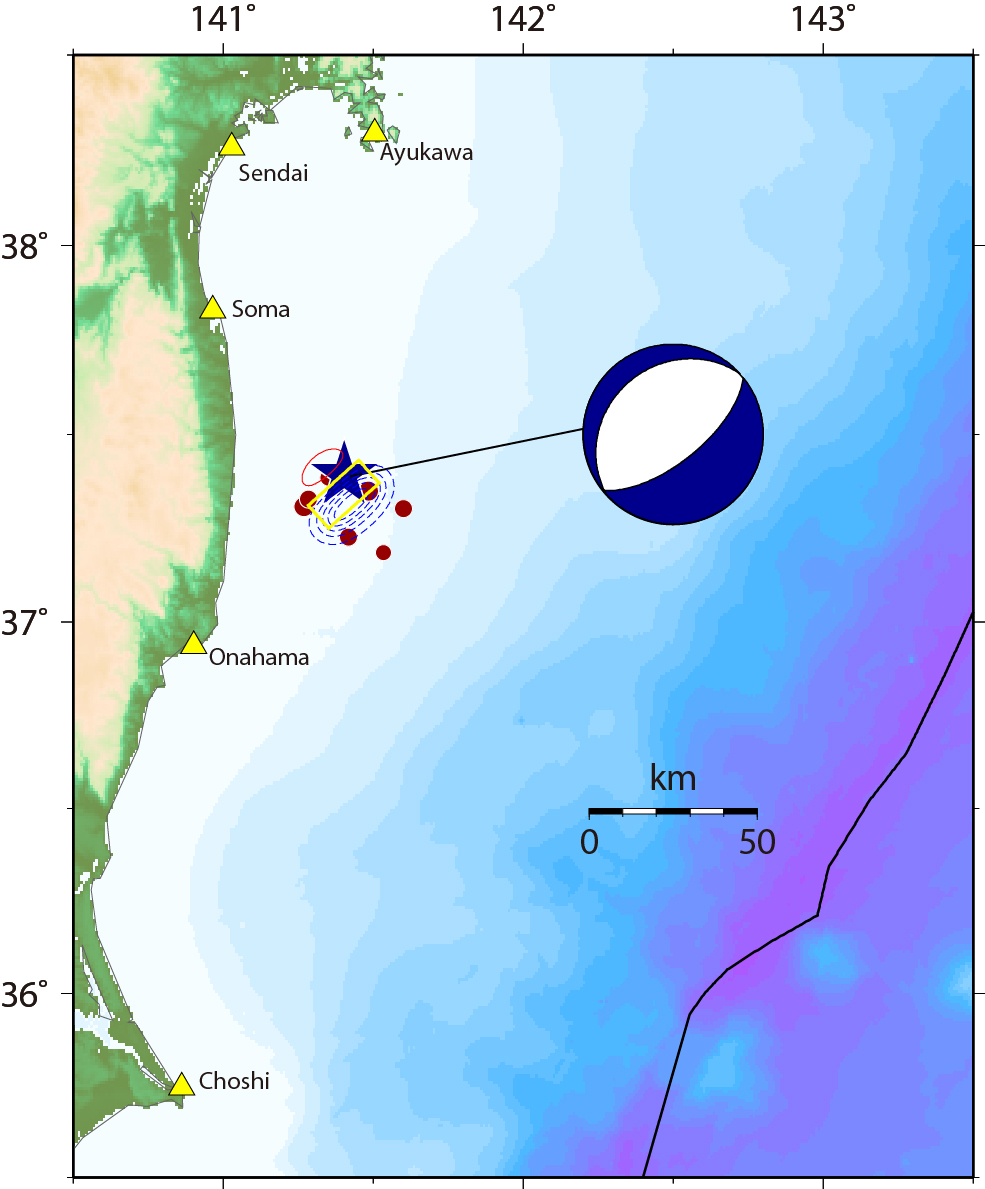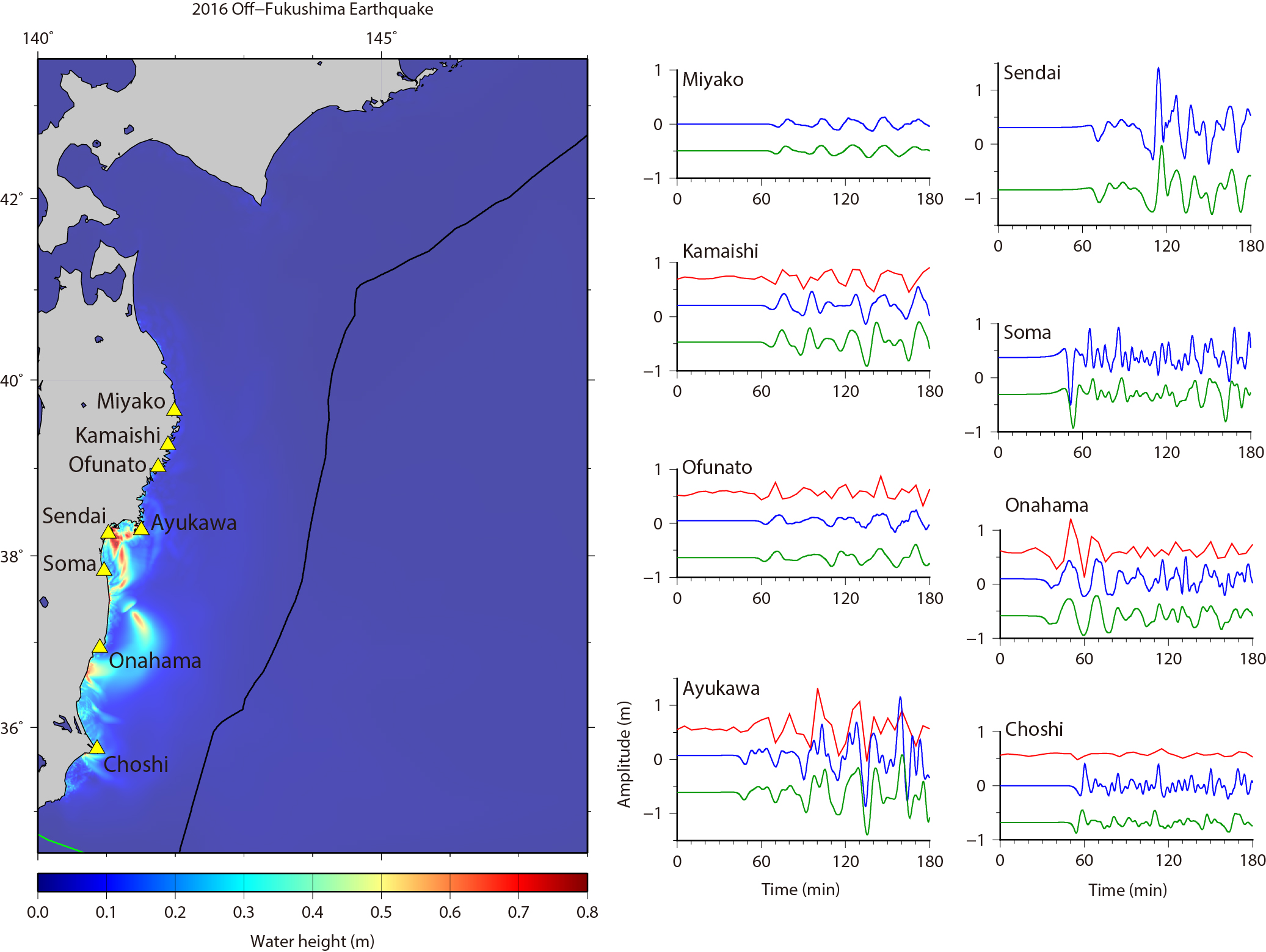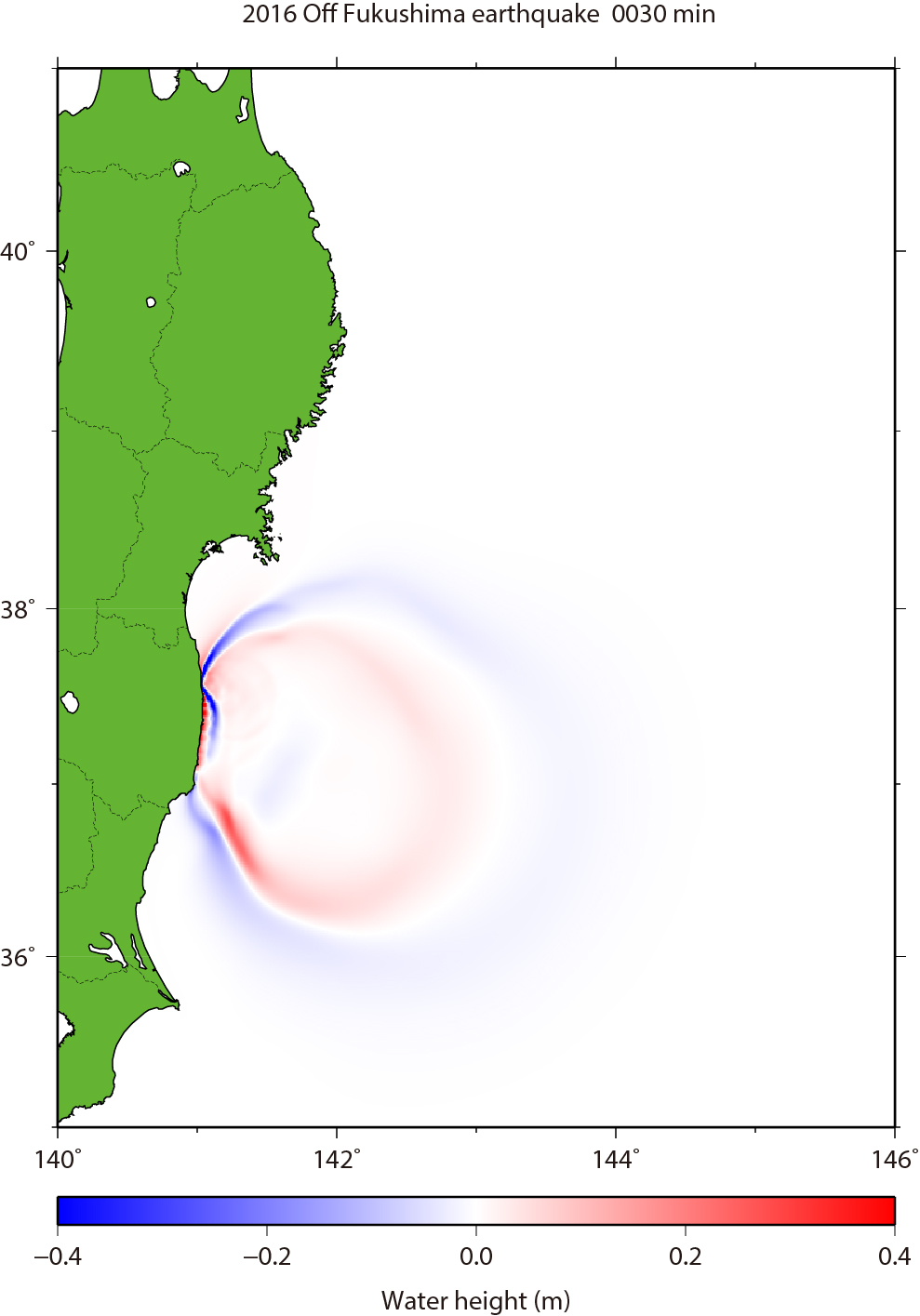Off-Fukushima Tsunami on Nov. 21, 2016

We have simulated the tsunami generated from the Off-Fukushima Japan earthquake (37.392°N, 141.403°E, depth=11.4km, M=6.9 at 20:59:49 UTC according to USGS) on November 21, 2016 (Fig. 1). The assumed tsunami sources are based on the USGS's W-phase moment tensor solution of the earthquake.

Case 1: strike = 51°, dip angle = 65º and slip angle = -89º.
Case 2: strike = 228º, dip angle = 25º and slip angle = -93º.
The fault size is 20 km × 10 km for both cases. Top depth of the fault are assumed to 3 and 6 km for Cases 1 and 2, respectively. An average slip on fault is 4 m. The seismic moment is 4.0 x 10**19 Nm (Mw = 7.0) assuming the regidity of 5 x 10**10 N/m**2.

As the initial conditions for tsunami, static deformations of the seafloor are calculated for a rectangular fault model [Okada, 1985] using the source models (Fig. 1). For the tide gauges along the coast, we used the bathymetry data of J-EGG500 with 12 arc-second grid interval. To calculate tsunami propagation, the linear shallow-water, or long-wave, equations were numerically solved by using a finite-difference method [Satake, 1995]. The maximum heights of simulated tsunami are shown with the calculated tsunami waveforms at coastal tide gauges in Fig. 2. We downloaded the tide gauge data from JCG's web site and compared the observed tsunami waveforms and simulated ones. We can see the tsunami propagation in the animation (Fig. 3).Fig.1 Tsunami source models of Case 1 (left) and Case 2(right).
The red contours indicate uplift with the contour interval of 0.2 m., while the blue contours indicate subsidence with the contour interval of 0.2 m.
Blue star and red circles show the mainshock and aftershocks, respectively, which were determined by USGS.
USGS's W-phase moment tensor solution and locations of tide gauges are also shown.Fig.2 Distribution of maximum height of the simulated tsunami (Case 1) and comparison of tsunami waveforms
Solid lines in red indicates the observed tsunami waveforms. The blue and green lines are synthtic tsunami waveforms for Case 1 and Case 2, respectively.Fig.3 Tsunami Propagation (Click to start animation)
The red color means that the water surface is higher than normal sea level, while the blue means lower.

 by Yushiro Fujii (IISEE, BRI) and Kenji Satake (ERI, Univ. Tokyo) References Okada, Y. (1985), Surface Deformation Due to Shear and Tensile Faults in a Half-Space, Bull. Seismol. Soc. Am., 75, 1135-1154. Satake, K. (1995), Linear and Nonlinear Computations of the 1992 Nicaragua Earthquake Tsunami, Pure and Appl. Geophys., 144, 455-470.

Last Updated on 2016/11/22